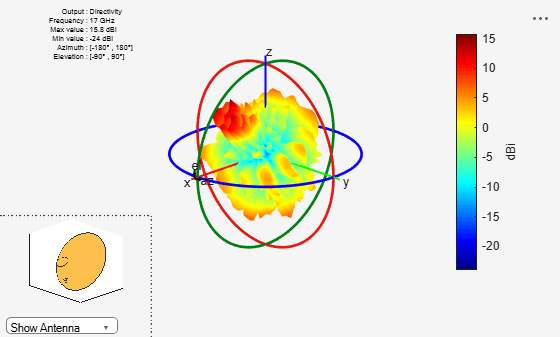# gregorianOffset

Create offset Gregorian antenna

## Description

The `gregorianOffset` object creates a offset Gregorian antenna. The offset Gregorian antenna is a parabolic antenna. It consists of feed antenna mounted off-axis to concave sub reflector and concave main reflector. The asymmetric arrangement of reflectors provides less blockage for waves redirected from main reflector. The advantage of these antennas is high gain, to reduce side-lobes and to improve cross polarization. The offset Gregorian antennas are used in satellite communication ground antennas, radar systems, and, radio telescopes, etc.## Creation

### Syntax

``ant = gregorianOffset``
``ant = gregorianOffset(Name,Value)``

### Description

example

````ant = gregorianOffset` creates a conical horn fed offset Gregorian antenna with dimensions for a resonant frequency of 17.76 GHz.```

example

````ant = gregorianOffset(Name,Value)` sets Properties using one or more name-value pairs. For example, ```ant = gregorianOffset('FocalLength', 0.04)``` creates an offset Gregorian antenna with the focal length of main reflector set to 40 mm.```

## Properties

expand all

Antenna type used as exciter, specified as an antenna or an array object.

Example: `'Exciter',dipole`

Example: `ant.Exciter = dipole`

Example: ```ant.Exciter = rectangularArray('invertedF')```

Radius of the main and sub reflector, specified as a two-element vector with each element unit in meters. The first element specifies the radius of the main reflector, and the second element specifies the radius of the sub reflector.

Example: `'Radius',[0.4 0.2]`

Example: `ant.Radius = [0.4 0.2]`

Data Types: `double`

Focal length of the main reflector, specified as a positive scalar integer in meters.

Example: `'FocalLength',0.0850`

Example: `ant.FocalLength = 0.0850`

Data Types: `double`

The distance between the main reflector and x=0 along X-axis, specified as a positive scalar integer in meters.

Example: `'MainReflectorOffset',0.8`

Example: `ant.MainReflectorOffset = 0.8`

Data Types: `double`

The spacing between the bottom edge of the main reflector and the top edge of the sub reflector along X-axis, specified as a positive scalar integer in meters.

Example: `'DualReflectorSpacing',0.8`

Example: `ant.DualReflectorSpacing = 0.8`

Data Types: `double`

Angle between the main reflector and the sub reflector co-ordinate systems, specified as a positive scalar integer in degrees.

Example: `'InterAxialAngle',8`

Example: `ant.InterAxialAngle = 8`

Data Types: `double`

Tilt angle of the reflectors, specified as a two-element vector with each element unit in degrees. The first element specifies the tilt of the main reflector, and the second element specifies the tilt of the sub reflector.

Note

You can use property BasisReflectorTilt to obtain initial value of tilt angles of reflectors with respect to reflector dimensions.

Example: `'ReflectorTilt',[60 20]`

Example: `ant.ReflectorTilt = [60 20]`

Data Types: `double`

Tilt angle of the antenna, specified as a scalar or vector with each element unit in degrees. For more information, see Rotate Antennas and Arrays.

Example: `'Tilt',90`

Example: `ant.Tilt = 90`

Example: `'Tilt',[90 90]`,```'TiltAxis',[0 1 0;0 1 1]``` tilts the antenna at 90 degrees about the two axes defined by the vectors.

Data Types: `double`

Tilt axis of the antenna, specified as:

• Three-element vector of Cartesian coordinates in meters. In this case, each coordinate in the vector starts at the origin and lies along the specified points on the X-, Y-, and Z-axes.

• Two points in space, each specified as three-element vectors of Cartesian coordinates. In this case, the antenna rotates around the line joining the two points in space.

• A string input describing simple rotations around one of the principal axes, 'X', 'Y', or 'Z'.

Example: `'TiltAxis',[0 1 0]`

Example: `'TiltAxis',[0 0 0;0 1 0]`

Example: `ant.TiltAxis = 'Z'`

Lumped elements added to the antenna feed, specified as a `lumpedElement` object handle. You can add a load anywhere on the surface of the antenna. By default, the load is at the feed. For more information, see `lumpedElement`.

Example: `'Load',lumpedelement`, where `lumpedelement` is the load added to the antenna feed.

Example: `ant.Load = lumpedElement('Impedance',75)`

Solver for antenna analysis, specified as the comma-separated pair consisting of `'SolverType'` and `'MoM-PO'` or `'MoM'` (Method of Moments) or `'FMM'` (Fast Multipole Method).

Example: `'SolverType'`,`'MOM'`

Data Types: `char`

## Object Functions

 `show` Display antenna, array structures or shapes `solver` Access FMM solver for electromagnetic analysis `axialRatio` Axial ratio of antenna `beamwidth` Beamwidth of antenna `charge` Charge distribution on antenna or array surface `current` Current distribution on antenna or array surface `design` Design prototype antenna or arrays for resonance around specified frequency `EHfields` Electric and magnetic fields of antennas; Embedded electric and magnetic fields of antenna element in arrays `impedance` Input impedance of antenna; scan impedance of array `mesh` Mesh properties of metal, dielectric antenna, or array structure `meshconfig` Change mesh mode of antenna structure `optimize` Optimize antenna or array using SADEA optimizer `pattern` Radiation pattern and phase of antenna or array; Embedded pattern of antenna element in array `patternAzimuth` Azimuth pattern of antenna or array `patternElevation` Elevation pattern of antenna or array `rcs` Calculate and plot radar cross section (RCS) of platform, antenna, or array `returnLoss` Return loss of antenna; scan return loss of array `sparameters` Calculate S-parameter for antenna and antenna array objects `vswr` Voltage standing wave ratio of antenna

## Examples

collapse all

Create an offset Gregorian dual-reflector antenna with default properties.

`ant = gregorianOffset`
```ant = gregorianOffset with properties: Exciter: [1x1 hornConical] Radius: [0.3000 0.0600] FocalLength: 0.2450 MainReflectorOffset: 0.2600 InterAxialAngle: 15 DualReflectorSpacing: 0.0450 ReflectorTilt: [55.9000 31.6000] Tilt: 0 TiltAxis: [1 0 0] Load: [1x1 lumpedElement] SolverType: 'MoM-PO' ```

View the antenna using the `show` function.

`show(ant)`Plot the radiation pattern of the offset Gregorian dual-reflector antenna at a frequency of 17 GHz.

`pattern(ant,17e9)`Create a circular array of rectangular spiral antennas.

```e = spiralRectangular; arr = circularArray('Element',e,'Radius',0.02);```

Create an offset Gregorian antenna with the circular array as the exciter.

`ant = gregorianOffset('Exciter',arr)`
```ant = gregorianOffset with properties: Exciter: [1x1 circularArray] Radius: [0.3000 0.0600] FocalLength: 0.2450 MainReflectorOffset: 0.2600 InterAxialAngle: 15 DualReflectorSpacing: 0.0450 ReflectorTilt: [55.9000 31.6000] Tilt: 0 TiltAxis: [1 0 0] Load: [1x1 lumpedElement] SolverType: 'MoM-PO' ```
`show(ant)`